Courses

# Questions with Answers: Probability Banking Exams Notes | EduRev

## CAT : Questions with Answers: Probability Banking Exams Notes | EduRev

The document Questions with Answers: Probability Banking Exams Notes | EduRev is a part of the CAT Course General Aptitude for GATE.
All you need of CAT at this link: CAT

Question 1:  One ticket is selected at random from 50 tickets numbered  0, 01, 02, ……, 49.   Then,   the probability that the sum of the digits on the selected ticket is 8, given that the product of these digits is zero equals

(a) 1/14
(b) 1/7
(c) 5/14
(d) 1/50

Explanation: (a)

Here we have

Let A be the event that sum of the digits on the selected ticket is 8

= {08,17, 26, 35, 44}

Let B be the event that the product of the digits is zero.

B = {00, 01, 02, 03, 04, 05, 06, 07, 08, 09, 10, 20, 30, 40}

∴ n(A∩B)=1 as only 08 is the number with sum 8 and product zero

n(B)=14

∴ required probabilty = P(A/B)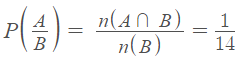Question 2: It is given that the events A and B are such that P(A) = ¼ , P(A|B) = ½ and P(B|A) = 2/3. Then P(B|A) = 2/3. Then P(B) is
(a) 1/2
(b) 1/6
(c) 1/3
(d) 2/3

Explanation: (c)

Given that P(A) = 1/4,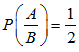and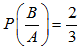we know,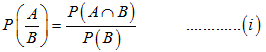and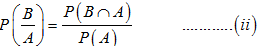Now, substitute the values in the formula, we get

(⅔ ) = P(B∩A)/ (¼)

P(B∩A) = (⅔). (¼)

P(B∩A) = ⅙

Substitute the values in (1), we get

P(A/B) = P(A∩B)/ P(B)

(½) = (⅙)/ P(B)

P(B) = (⅙). (2/1)

P(B) = 1/3

Question 3: A die is thrown. Let A be the event that the number obtained is greater than 3. Let B be the event that the number obtained is less than 5. Then P (AUB) is
(a) 2/5
(b) 3/5
(c) 0
(d) 1

Explanation: (d)

Since,

A= number>3

A {4,5,6}

and B = number< 5

B = {1,2,3,4}

∴ A∩B = {4}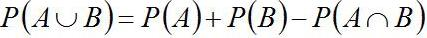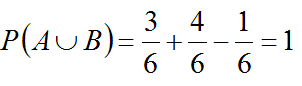Question 4: A pair of fair dice is thrown independently three times. The probability of getting a total of exactly 9 twice is

(a) 1/729
(b) 8/9
(c) 8/729
(d) 8/243

Explanation: (d)

When two dice are thrown, the total number of sample space is 36

Probability of getting a total of 9 in a single throw

4/36 = 1/9

Hence, the probability of getting a score of exactly 9 twice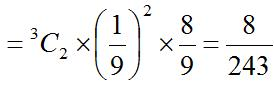Question 5: Two aeroplanes I and II bomb a target in succession. The probabilities of I and II scoring a hit correctly are 0.3 and 0.2, respectively. The second plane will bomb only if the first misses the target. The probability that the target is hit by the second plane, is
(a) 0.06
(b) 0.14
(c) 0.32
(d) 0.7

Explanation: (c)

Let the events,

A = 1st aeroplane hit the target

B =2nd aeroplane hit the target

And their corresponding probabilities are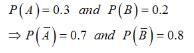Required Probability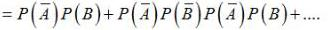= (0.7)(0.2) + (0.7)(0.8)(0.7)(0.2)+(0.7)(0.8)(0.7)(0.8)(0.7)(0.2) + ....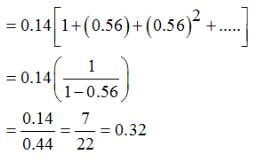Offer running on EduRev: Apply code STAYHOME200 to get INR 200 off on our premium plan EduRev Infinity!

## General Aptitude for GATE

60 videos|47 docs|108 tests

,

,

,

,

,

,

,

,

,

,

,

,

,

,

,

,

,

,

,

,

,

;